Next: Track Fitting under the Up: Track Fitting in r- Previous: Pivot Transformation in Vacuum

## Inclusion of Multiple Scattering

When the pivot is taken at the point of multiple scattering, only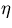is affected among the track parameters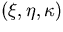. The scattering angle in the r-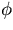plane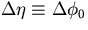has a variance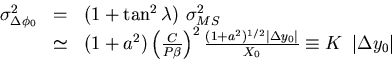(47)
with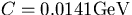, P and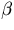are the momentum and the velocity of the particle, and X0 is the radiation length of the material. This modifies (E)22 and Eq.3.2.9 now becomes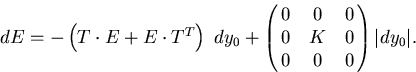(48)
Notice that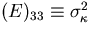is not affected by the pivot transformation, which allows us to readily integrate the above equation. The solution of the above equation can be written in the form: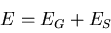where EG is the general solution for K = 0, while ES is a special solution of Eq.3.2.12. It is easily verified that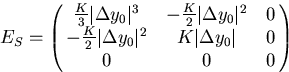(49)
satisfies Eq.3.2.12. It is also easy to show that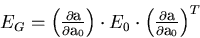(50)
with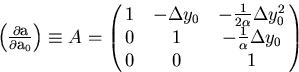(51)
is the general solution of the homogeneous equation (K=0).

In summary, the propagated error matrix at the point which is outside the area of coordinate measurements is the sum:(52)
where EG is the propagated error matrix without taking multiple scattering into account (Eqs.3.2.15 and 3.2.16) and ES is the contribution from multiple scattering (Eq.3.2.14).

Now that we have established the machinary to move the pivot to anywhere in the detector system, it is easy to implement the information from external tracking devices. Assume that we have an extra coordinate measurement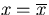at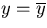. Then we take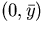as our new pivot and minimize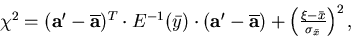(53)
which is a simple matrix inversion. The corresponding error matrix can also be readily obtained from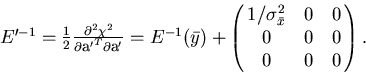(54)
If there are more than one extra measurements, we can retransform E' to the next and do the same.

If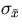is negligibly small, we might even include this as a constraint on the track parameter. In this case, the track becomes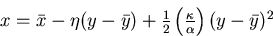(55)
which has only two parameters: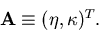The error matrix for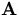is given by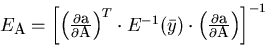(56)
with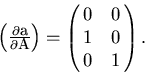(57)Next: Track Fitting under the Up: Track Fitting in r- Previous: Pivot Transformation in Vacuum
Keisuke Fujii
12/4/1998